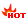华图教育

+ 关注
QQ群

# 2021国家公务员考试各部门综合成绩计算方法|总成绩计算方式汇总

2020-08-06 14:09:31 国家公务员考试网 http://gx.huatu.com/guojia/ 文章来源：国家公务员考试网【导读】华图国家公务员考试网同步国家公务员考试网发布：2021国家公务员考试各部门综合成绩计算方法|总成绩计算方式汇总,更多关于国家公务员,广西公务员,公务员考试的内容请关注广西国家公务员考试网,以及广西华图微信公众号(gxhuatu)和国考交流群:928698228）,获取更多招考信息和备考资料。

2021国家公务员考试总成绩计算公式将会同步国家各部门官方及时更新，请收藏本站，以下为历年国家公务员考试综合成绩计算方法，供各位考生查阅了解：

历年国家公务员考试各部门综合成绩计算方法|总成绩计算方法分两种情况：

 部门 综合成绩计算方法 中国科协 综合成绩(无专业科目考试) =(笔试总成绩÷2)×50% + 面试成绩×50% 综合成绩(有专业科目考试) =(笔试总成绩÷2)×50% + 面试成绩×35%+专业科目考试成绩×15% 中央组织部机关 公共科目笔试、专业能力测试、面试成绩按5:1.5:3.5比例加权计算综合成绩.考试结束后,电话通知考生个人综合成绩。 财政部 综合成绩=(笔试总成绩÷2)×50% + 面试成绩×50% 中国贸促会 综合成绩=公共科目笔试成绩×50%+面试×35%+中文笔试×5%+外语笔试×5%+外语口试×5%。 住房和城乡建设部 综合成绩 =(笔试总成绩÷2)×50% + 面试成绩×50% 国家移民管理局 综合成绩=笔试合成成绩(行政职业能力测验占40%,申论占30%,公安类专业科目考试占30%)×50% + 面试成绩×50% 公安部 综合成绩(无专业能力测试)=笔试合成分数×50% + 面试成绩×50% 综合成绩(有专业能力测试)=笔试合成分数×50% + 面试成绩×35%+专业能力测试成绩×15% 应急管理部 综合成绩(无专业能力测试) =(笔试总成绩÷2)X50% + 面试成绩X50% 综合成绩(有专业能力测试) =(笔试总成绩÷2)X50% + 面试成绩X35%+专业能力测试成绩X15% 国家能源局 综合成绩=(笔试总成绩÷2)×50% + 面试成绩×35%+专业能力测试成绩×15% 司法部燕城监狱 综合成绩 =(笔试总成绩÷2)×50% + 面试成绩×50% 人力资源和社会保障部 综合成绩(无专业能力测试)=(笔试成绩÷2)×50%+面试成绩×50% 国务院发展研究中心 综合成绩=(笔试总成绩÷2)×50% + 面试成绩×35%+专业能力测试×15% 生态环境部派出机构 综合成绩 =(笔试总成绩÷2)×50% + 面试成绩×50% 中国人民对外友好协会 公共科目笔试成绩(小语种按公共科目笔试和语言测试的合成成绩)占50%、专业考试成绩占15%、面试成绩占35% 最高人民法院 综合成绩=(笔试总成绩÷2)×50% + 面试成绩×50%。 中国人民银行 综合成绩=公务员公共科目笔试成绩÷2×50%+面试成绩×50% 退役军人事务部 综合成绩=(笔试总成绩÷2)×50%+面试成绩×50% 中国外文局 综合成绩=(笔试总成绩÷2)*50% +面试成绩*35%+专业能力测试成绩*15% 中国气象局 综合成绩=(笔试总成绩÷2)×50% + 面试成绩×50% 中华全国供销合作总社 综合成绩=(笔试总成绩÷2)×50%+面试成绩×50% 生态环境部 综合成绩(无专业能力测试) =(笔试总成绩÷2)X50% + 面试成绩X50% 综合成绩(有专业能力测试) =(笔试总成绩÷2)X50% + 面试成绩X35%+专业能力测试成绩X15% 工业和信息化部 公共科目笔试总成绩(换算为百分制)和面试成绩各占50% 外交部 公共科目笔试成绩(日语等8个非通用语职位笔试合成成绩)占50%，面试成绩占30%，专业能力测试成绩占20%。 全国人大机关 一、综合成绩(无专业能力测试)=(笔试总成绩÷2)×50%+面试成绩×50% 二、综合成绩(有外语专业能力测试)=(笔试总成绩÷2)×50%+面试成绩×35%+外语专业能力测试成绩×15% 司法部 综合成绩(无专业科目考试) =(笔试总成绩÷2)×50% + 面试成绩×50% 中国浦东干部学院 综合成绩(无专业能力测试)=(笔试总成绩÷2)×50% + 面试成绩×50% 外交学会 公共科目笔试成绩占50%，面试成绩占35%，专业能力测试成绩占15% 农工党中央机关 一、综合成绩(无专业能力测试) =(笔试总成绩÷2)X50% + 面试成绩X50% 二、综合成绩(有专业能力测试) =(笔试总成绩÷2)X50% + 面试成绩X35%+专业能力测试成绩X15% 科技部 综合成绩=(笔试总成绩÷2)×50%+面试成绩×35%+专业能力测试成绩×15% 国家体育总局 一、综合成绩(无专业能力测试)=(笔试总成绩÷2)X50% +面试成绩X50% 二、综合成绩(有专业能力测试) =(笔试总成绩÷2)X50% + 面试成绩X35%+专业能力测试成绩X15% 中国延安干部学院 综合成绩(无专业能力测试) =(笔试总成绩÷2)×50% + 面试成绩×50% 中国文联 一、综合成绩(无专业能力测试)=(笔试总成绩÷2)×50%+面试成绩×50% 二、综合成绩(有专业能力测试)=(笔试总成绩÷2)×50%+面试成绩×35%+专业能力测试成绩×15% 中央纪委国家监委 一、综合成绩(无专业能力测试)=(笔试总成绩÷2)×50% + 面试成绩×50% 二、综合成绩(有专业能力测试)=(笔试总成绩÷2)×50% + 面试成绩×35%+专业科目考试成绩×15%。 中央档案馆国家档案局 一、综合成绩(无专业能力测试)=(笔试总成绩÷2)×50%+面试成绩×50%；二、综合成绩(有专业能力测试)=(笔试总成绩÷2)×50%+面试成绩×35%+专业能力测试成绩×15% 国家统计局 综合成绩=(笔试总成绩÷2)×50% + 面试成绩×50%。 中央社会主义学院 综合成绩=(笔试总成绩÷2)×50% + 面试成绩×50% 水利部松辽水利委员 综合成绩=(笔试总成绩÷2)×50% + 面试成绩×50% 国务院参事室 综合成绩=(笔试总成绩÷2)×50% + 面试成绩×50% 中华全国台湾同胞联谊会 综合成绩=(笔试总成绩÷2)×50%+面试成绩×40%+专业能力测试成绩×10% 中国作家协会 一、办公厅职位综合成绩=(笔试总成绩÷2)×50% + 面试成绩×35%+专业能力测试×15% 二、社会联络部职位综合成绩=(笔试总成绩÷2)×50% + 面试成绩×50% 民盟中央 综合成绩=(笔试总成绩÷2)×50% + 面试成绩×50% 国家民族事务委员会 一、无专业能力测试的岗位：综合成绩=(笔试总成绩÷2)×50% + 面试成绩×50% 二、有专业能力测试的岗位：综合成绩=(笔试总成绩÷2)×50% + 面试成绩×35%+专业能力测试成绩×15% 国家发展改革委 综合成绩[(笔试总成绩÷2)×50%+面试成绩×50%] 国家文物局 一、综合成绩计算: 综合成绩(无专业能力测试) =(笔试总成绩÷2)X50% + 面试成绩X50% 二、综合成绩(有专业能力测试) =(笔试总成绩÷2)X50% + 面试成绩X35%+专业能力测试成绩X15% 文化和旅游部 综合成绩=(笔试总成绩÷2)×50% + (结构化面试成绩+无领导小组讨论成绩)÷2×35%+专业能力测试成绩×15%。 国家卫生健康委 综合成绩=(笔试总成绩÷2)X50%+面试成绩X50% 银保监会人事部 公共科目笔试(行政职业能力测验、申论)总成绩(按1:1折算为百分制后)占25%，专业科目笔试成绩占25%，面试成绩占50% 水利部珠江水利委员会 综合成绩 =(笔试总成绩÷2)X50% + 面试成绩X50% 商务部 公共科目笔试成绩(非通用语含专业初试成绩)占50%，专业能力测试成绩占15%，面试成绩占35% 农业农村部 综合成绩=(笔试总成绩÷2)×50%+面试成绩×50% 国家中医药管理局 综合成绩=(笔试总成绩÷2)×50% + 面试成绩×50% 国家煤矿安全监察系统 综合成绩=(笔试总成绩÷2)×50% + 面试成绩×50% 民建中央 综合成绩(无专业能力测试) =(笔试总成绩÷2)X50% + 面试成绩X50% 中央政府驻澳门联络办公室 综合成绩 =(笔试总成绩÷2)X50% + 面试成绩X35%+专业能力测试成绩X15% 交通运输部 综合成绩(无专业能力测试) =(笔试总成绩÷2)×50% + 面试成绩×50%； 综合成绩(有专业能力测试) =(笔试总成绩÷2)×50% + 面试成绩×35%+专业能力测试成绩×15% 民进中央 综合成绩(无专业能力测试) =(笔试总成绩÷2)X50% + 面试成绩X50% 中央办公厅 综合成绩(无专业科目考试)=(笔试总成绩÷2)×50% +面试成绩×50%； 综合成绩(有专业科目考试)=(笔试总成绩÷2)×50% +面试成绩×35%+专业科目考试成绩×15% 中国地质调查局 综合成绩=(笔试总成绩÷2)×50%+面试成绩×50% 中国民航空中警察系统 综合成绩=合成笔试成绩×50% + 面试成绩×50% 国家邮政局系统 综合成绩=(笔试总成绩÷2)×50% + 面试成绩×50% 水利部长江水利委员会 综合成绩(无专业能力测试) =(笔试总成绩÷2)X50% + 面试成绩X50% 中国残疾人联合会 综合成绩=(笔试总成绩÷2)×50% +面试成绩×50% 国家机关事务管理局 综合成绩=(笔试总成绩÷2)×50%+面试成绩×50% 中国地震局 综合成绩=（笔试总成绩÷2）X50% + 面试成绩X50% 水利部太湖流域管理局 综合成绩(无专业能力测试) =(笔试总成绩÷2)×50% + 面试成绩×50% 中国侨联 综合成绩=(笔试总成绩÷2)×50% + 面试成绩×50% 交通运输部系统(海事局) 综合成绩=笔试÷2×50%+面试成绩×50% 全国税务系统 综合成绩=(笔试总成绩÷2)×50%+面试成绩×50% 中国地方志指导小组办公室 综合成绩(无专业能力测试) =(笔试总成绩÷2)X50% + 面试成绩X50%
 2021年国家公务员面试信息汇总 时间安排 招考信息 成绩2021国考成绩查询入口| 成绩排名查询 分数国考笔试合格分数线丨历年入面分数线 面试 2021国考面试名单下载丨入面名单查询 课程 2021国考面试课程丨网络课程 资料 2021国考面试礼包丨历年面试题目 讲座 2021国考面试解读峰会丨面试1对1测评

以上为2021国家公务员考试各部门综合成绩计算方法|总成绩计算方式汇总的内容，更多关于国家公务员,广西公务员,公务员考试的信息请关注广西国家公务员考试网/广西人事考试网

（编辑：广西-华华）Q群刷题备考

2020区考交流群：928698228在线客服×• 南宁市
• 大学城分校
• 桂林市
• 荔浦市
• 百色市
• 隆林县
• 玉林市
• 北海市
• 钦州市
• 贵港市
• 桂平市
• 崇左市
• 防城港市
• 柳州市
• 河池市
• 梧州市
• 岑溪市
• 来宾市
• 忻城县
• 贺州市
• 华图总部